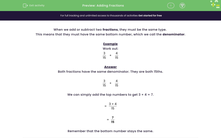### Comprehensive & curriculum aligned

In this worksheet, students add fractions with the same denominator.Key stage:  KS 2

Curriculum topic:   Number: Fractions and Decimals

Difficulty level:#### Worksheet Overview

When we add or subtract two fractions, they must be the same type.

This means that they must have the same bottom number, which we call the denominator.

Example

Work out:

 3 + 4 15 15

Both fractions have the same denominator. They are both 15ths.

 3 + 4 15 15

We can simply add the top numbers to get 3 + 4 = 7.

 = 3 + 4 15

 = 7 15

Remember that the bottom number stays the same.

### What is EdPlace?

We're your National Curriculum aligned online education content provider helping each child succeed in English, maths and science from year 1 to GCSE. With an EdPlace account you’ll be able to track and measure progress, helping each child achieve their best. We build confidence and attainment by personalising each child’s learning at a level that suits them.

Get started